# Labeled Image Functions¶

Labeled images are integer images where the values correspond to different regions. I.e., region 1 is all of the pixels which have value 1, region two is the pixels with value 2, and so on. By convention, region 0 is the background and often handled differently.

## Labeling Images¶

New in version 0.6.5.

The first step is obtaining a labeled function from a binary function:

```import mahotas as mh
import numpy as np
from pylab import imshow, show

regions = np.zeros((8,8), bool)

regions[:3,:3] = 1
regions[6:,6:] = 1
labeled, nr_objects = mh.label(regions)

imshow(labeled, interpolation='nearest')
show()
```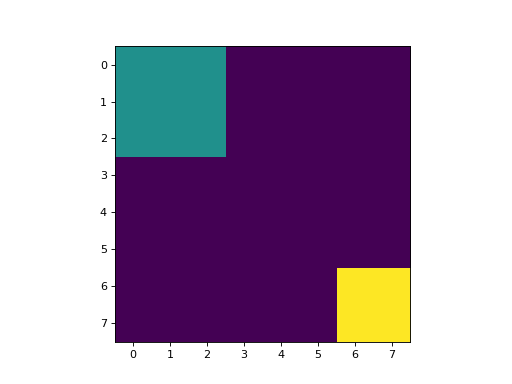This results in an image with 3 values:

1. background, where the original image was 0
2. for the first region: (0:3, 0:3);
3. for the second region: (6:, 6:).

There is an extra argument to `label`: the structuring element, which defaults to a 3x3 cross (or, 4-neighbourhood). This defines what it means for two pixels to be in the same region. You can use 8-neighbourhoods by replacing it with a square:

```labeled,nr_objects = mh.label(regions, np.ones((3,3), bool))
```

We can now collect a few statistics on the labeled regions. For example, how big are they?

```sizes = mh.labeled.labeled_size(labeled)
print('Background size', sizes)
print('Size of first region: {}'.format(sizes))
```

This size is measured simply as the number of pixels in each region. We can instead measure the total weight in each area:

```array = np.random.random_sample(regions.shape)
sums = mh.labeled_sum(array, labeled)
print('Sum of first region: {}'.format(sums))
```

## Filtering Regions¶

New in version 0.9.6: `remove_regions` & `relabel` were added.

Here is a slightly more complex example. The full code is in the demos directory as `nuclear.py`. We are going to use this image, a fluorescent microscopy image from a nuclear segmentation benchmark

This image is available as `mahotas.demos.nuclear_image()`

```import mahotas as mh
import mahotas.demos
import numpy as np
from pylab import imshow, show

f = mh.demos.nuclear_image()
f = f[:,:,0]
imshow(f)
show()
```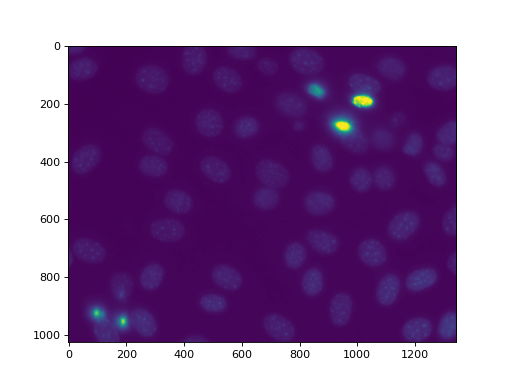First we perform a bit of Gaussian filtering and thresholding:

```f = mh.gaussian_filter(f, 4)
f = (f> f.mean())
```

(Without the Gaussian filter, the resulting thresholded image has very noisy edges. You can get the image in the `demos/` directory and try it out.)

```f = mh.gaussian_filter(f, 4)
f = (f> f.mean())
imshow(f)
show()
```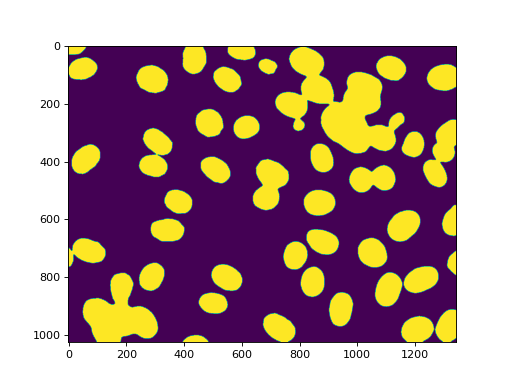Labeling gets us all of the nuclei:

```labeled, n_nucleus  = mh.label(f)
print('Found {} nuclei.'.format(n_nucleus))
```
```labeled, n_nucleus  = mh.label(f)
print('Found {} nuclei.'.format(n_nucleus))
imshow(labeled)
show()
```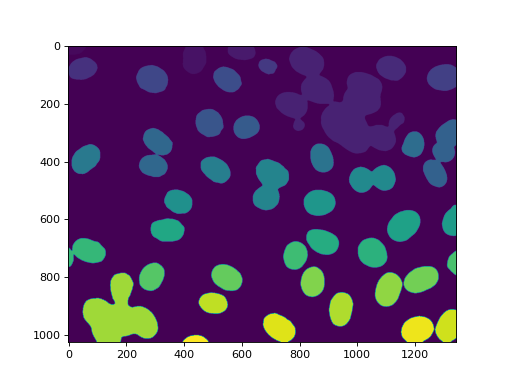`42` nuclei were found. None were missed, but, unfortunately, we also get some aggregates. In this case, we are going to assume that we wanted to perform some measurements on the real nuclei, but are willing to filter out anything that is not a complete nucleus or that is a lump on nuclei. So we measure sizes and filter:

```sizes = mh.labeled.labeled_size(labeled)
too_big = np.where(sizes > 10000)
labeled = mh.labeled.remove_regions(labeled, too_big)
```
```sizes = mh.labeled.labeled_size(labeled)
too_big = np.where(sizes > 10000)
labeled = mh.labeled.remove_regions(labeled, too_big)
imshow(labeled)
show()
```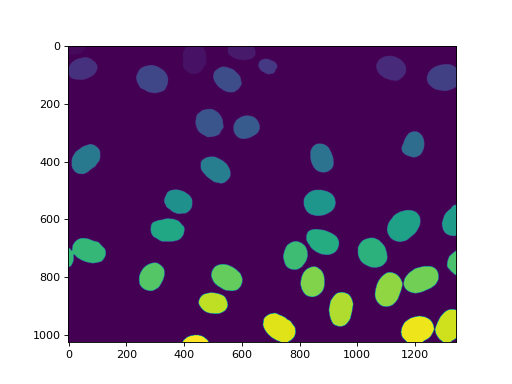We can also remove the region touching the border:

```labeled = mh.labeled.remove_bordering(labeled)
```
```labeled = mh.labeled.remove_bordering(labeled)
imshow(labeled)
show()
```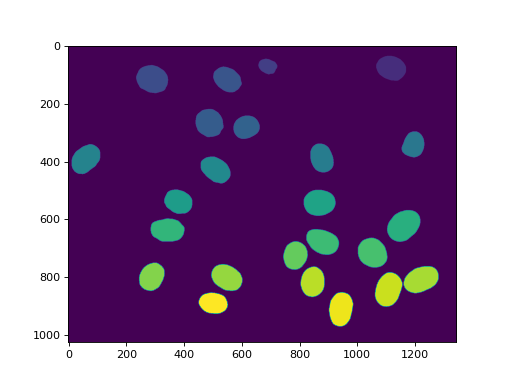This array, `labeled` now has values in the range `0` to `n_nucleus`, but with some values missing (e.g., if region `7` was one of the ones touching the border, then `7` is not used in the labeling). We can `relabel` to get a cleaner version:

```relabeled, n_left = mh.labeled.relabel(labeled)
print('After filtering and relabeling, there are {} nuclei left.'.format(n_left))
```

Now, we have `24` nuclei and `relabeled` goes from `0` (background) to `24`.

```relabeled, n_left = mh.labeled.relabel(labeled)
print('After filtering and relabeling, there are {} nuclei left.'.format(n_left))
imshow(relabeled)
show()
```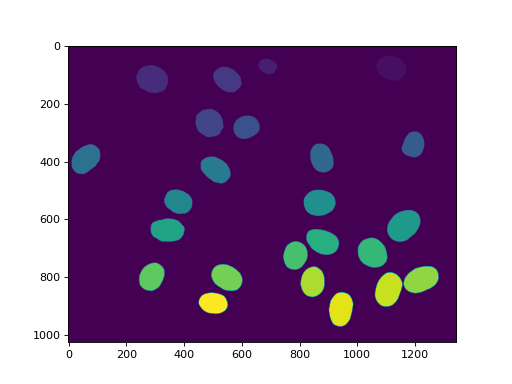In mahotas after version `1.4`, we can even make many of the same operations with a single call to `mh.labeled.filter_labeled`:

```relabeled,n_left = mh.labeled.filter_labeled(labeled, remove_bordering=True, max_size=10000)
```

## Borders¶

A border pixel is one where there is more than one region in its neighbourhood (one of those regions can be the background).

You can retrieve border pixels with either the `borders()` function, which gets all the borders or the `border()` (note the singular) which gets only the border between a single pair of regions. As usual, what neighbour means is defined by a structuring element, defaulting to a 3x3 cross.

## API Documentation¶

The `mahotas.labeled` submodule contains the functions mentioned above. `label()` is also available as `mahotas.label`.

`mahotas.labeled.``bbox`(f, as_slice=False)

Bounding boxes of all objects in a labeled array.

After:

```bboxes = mh.labeled.bbox(f)
```

`bboxes` will contain the bounding box of `(f == 34)`.

Parameters: f : integer ndarray as_slice : boolean, optional Whether to return slice objects instead of integer coordinates (default: False). bboxes : ndarray

`mh.bbox`
the binary version of this function
`mahotas.labeled.``borders`(labeled, Bc={3x3 cross}, out={np.zeros(labeled.shape, bool)})

Compute border pixels

A pixel is on a border if it has value i and a pixel in its neighbourhood (defined by Bc) has value j, with `i != j`.

Parameters: labeled : ndarray of integer type input labeled array Bc : structure element, optional out : ndarray of same shape as labeled, dtype=bool, optional where to store the output. If `None`, a new array is allocated mode : {‘reflect’, ‘nearest’, ‘wrap’, ‘mirror’, ‘constant’ [default], ‘ignore’} How to handle borders border_img : boolean ndarray Pixels are True exactly where there is a border in labeled
`mahotas.labeled.``border`(labeled, i, j, Bc={3x3 cross}, out={np.zeros(labeled.shape, bool)}, always_return=True)

Compute the border region between i and j regions.

A pixel is on the border if it has value i (or j) and a pixel in its neighbourhood (defined by Bc) has value j (or i).

Parameters: labeled : ndarray of integer type input labeled array i : integer j : integer Bc : structure element, optional out : ndarray of same shape as labeled, dtype=bool, optional where to store the output. If `None`, a new array is allocated always_return : bool, optional if false, then, in the case where there is no pixel on the border, returns `None`. Otherwise (the default), it always returns an array even if it is empty. border_img : boolean ndarray Pixels are True exactly where there is a border between i and j in labeled
`mahotas.labeled.``bwperim`(bw, n=4)

Find the perimeter of objects in binary images.

A pixel is part of an object perimeter if its value is one and there is at least one zero-valued pixel in its neighborhood.

By default the neighborhood of a pixel is 4 nearest pixels, but if n is set to 8 the 8 nearest pixels will be considered.

Parameters: bw : ndarray A black-and-white image (any other image will be converted to black & white) n : int, optional Connectivity. Must be 4 or 8 (default: 4) mode : {‘reflect’, ‘nearest’, ‘wrap’, ‘mirror’, ‘constant’ [default], ‘ignore’} How to handle borders perim : ndarray A boolean image

`borders`
function This is a more generic function
`mahotas.labeled.``filter_labeled`(labeled, remove_bordering=False, min_size=None, max_size=None)

Filter labeled regions based on a series of conditions

New in version 1.4.1.

Parameters: labeled : labeled array remove_bordering : bool, optional whether to remove regions that touch the border min_size : int, optional Minimum size (in pixels) of objects to keep (default is no minimum) max_size : int, optional Maximum size (in pixels) of objects to keep (default is no maximum) filtered : labeled array nr : int number of new labels
`mahotas.labeled.``label`(array, Bc={3x3 cross}, output={new array})

Label the array, which is interpreted as a binary array

This is also called connected component labeled, where the connectivity is defined by the structuring element `Bc`.

Parameters: array : ndarray This will be interpreted as binary array Bc : ndarray, optional This is the structuring element to use out : ndarray, optional Output array. Must be a C-array, of type np.int32 labeled : ndarray Labeled result nr_objects : int Number of objects
`mahotas.labeled.``labeled_sum`(array, labeled, minlength=None)

Labeled sum. sum will be an array of size `labeled.max() + 1`, where `sum[i]` is equal to `np.sum(array[labeled == i])`.

Parameters: array : ndarray of any type labeled : int ndarray Label map. This is the same type as returned from `mahotas.label()` minlength : int, optional Minimum size of return array. If labeled has fewer than `minlength` regions, 0s are added to the result. (optional) sums : 1-d ndarray of `array.dtype`
`mahotas.labeled.``labeled_max`(array, labeled, minlength=None)

Labeled minimum. `mins` will be an array of size `labeled.max() + 1`, where `mins[i]` is equal to `np.min(array[labeled == i])`.

Parameters: array : ndarray of any type labeled : int ndarray Label map. This is the same type as returned from `mahotas.label()` mins : 1-d ndarray of `array.dtype`
`mahotas.labeled.``labeled_size`(labeled)

Equivalent to:

```for i in range(...):
sizes[i] = np.sum(labeled == i)
```

but, naturally, much faster.

Parameters: labeled : int ndarray sizes : 1-d ndarray of int

`mahotas.fullhistogram`
almost same function by another name (the only

`difference`

`mahotas.labeled.``relabel`(labeled, inplace=False)

Relabeling ensures that `relabeled` is a labeled image such that every label from 1 to `relabeled.max()` is used (0 is reserved for the background and is passed through).

Example:

```labeled,n = label(some_binary_map)
for region in xrange(n):
if not good_region(labeled, region + 1):
# This deletes the region:
labeled[labeled == (region + 1)] = 0
relabel(labeled, inplace=True)
```
Parameters: relabeled : ndarray of int A labeled array inplace : boolean, optional Whether to perform relabeling inplace, erasing the values in `labeled` (default: False) relabeled: ndarray nr_objs : int Number of objects

`label`
function
`mahotas.labeled.``is_same_labeling`(labeled0, labeled1)

Checks whether `labeled0` and `labeled1` represent the same labeling (i.e., whether they are the same except for a possible change of label values).

Note that the background (value 0) is treated differently. Namely

is_same_labeling(a, b) implies np.all( (a == 0) == (b == 0) )

Parameters: labeled0 : ndarray of int A labeled array labeled1 : ndarray of int A labeled array same : bool True if the labelings passed as argument are equivalent

`label`
function
`relabel`
function
`mahotas.labeled.``perimeter`(bwimage, n=4, mode="constant")

Calculate total perimeter of all objects in binary image.

Parameters: bwimage : array binary image n : int, optional passed to `bwperim` as is mode : str, optional passed to `bwperim` as is p : float total perimeter of all objects in binary image

`bwperim`
function Finds the perimeter region

References

 [R1] K. Benkrid, D. Crookes. Design and FPGA Implementation of a Perimeter Estimator. The Queen’s University of Belfast. http://www.cs.qub.ac.uk/~d.crookes/webpubs/papers/perimeter.doc
`mahotas.labeled.``remove_bordering`(labeled, rsize=1, out={np.empty_like(im)})

Remove objects that are touching the border.

Pass `labeled` as `out` to achieve in-place operation.

Parameters: labeled : ndarray Labeled array rsize : int or tuple, optional Minimum distance to the border (in Manhatan distance) to allow an object to survive. May be int or tuple with len == labeled.ndim. out : ndarray, optional If `im` is passed as `out`, then it operates inline. slabeled : ndarray Subset of `labeled`
`mahotas.labeled.``remove_regions`(labeled, regions, inplace=False)

removed = remove_regions(labeled, regions, inplace=False):

Removes the regions in `regions`. If an elementwise `in` operator existed, this would be equivalent to the following:

```labeled[ labeled element-wise-in regions ] = 0
```

This function does not relabel its arguments. You can use the `relabel` function for that:

```removed = relabel(remove_regions(labeled, regions))
```

Or, saving one image allocation:

```removed = relabel(remove_regions(labeled, regions), inplace=True)
```

This is the same, but reuses the memory in the relabeling operation.

Parameters: relabeled : ndarray of int A labeled array regions : sequence of int These regions will be removed inplace : boolean, optional Whether to perform removal inplace, erasing the values in `labeled` (default: False) removed : ndarray

`relabel`
function After removing unecessary regions, it is often a good idea to relabel your label image.
`mahotas.labeled.``remove_regions_where`(labeled, conditions, inplace=False)

Remove regions based on a boolean array

A region is removed if `conditions[region-id]` evaluates true.

This function does not relabel its arguments. You can use the `relabel` function for that:

```removed = relabel(remove_regions_where(labeled, conditions))
```

Or, saving one image allocation:

```removed = relabel(remove_regions(labeled, conditions), inplace=True)
```

This is the same, but reuses the memory in the relabeling operation.

`remove_regions`